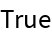#Function Repository Resource:

# SupplementaryAngle

Compute the geometric supplement to a given angle

Contributed by: Paco Jain (Wolfram Research)
 ResourceFunction["SupplementaryAngle"][θ] gives the supplement to the angle of θ.

## Details and Options

ResourceFunction["SupplementaryAngle"] takes all angles to be measured in radians.
The supplement of an angle θ is defined to be the angle that sums with θ to form a straight angle. In other words, π-θ.

## Examples

### Basic Examples

Compute the supplement of an angle:

 In:=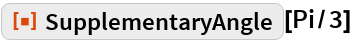Out=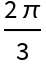### Scope

SupplementaryAngle works directly on Quantity expressions with units of "Radians":

 In:=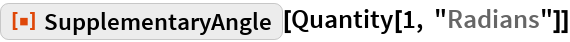Out=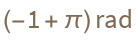### Properties and Relations

SupplementaryAngle will remain unevaluated for non-numeric input:

 In:=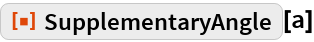Out=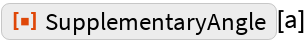SupplementaryAngle will return a negative result if the input angle is greater than π:

 In:=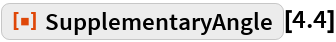Out=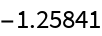For negative input, SupplementaryAngle returns a result greater than π:

 In:=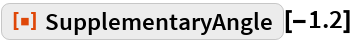Out=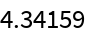For numeric input, SupplementaryAngle is its own inverse:

 In:=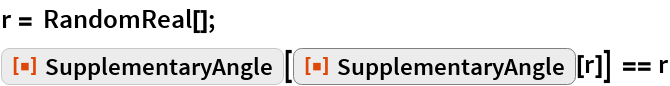Out=